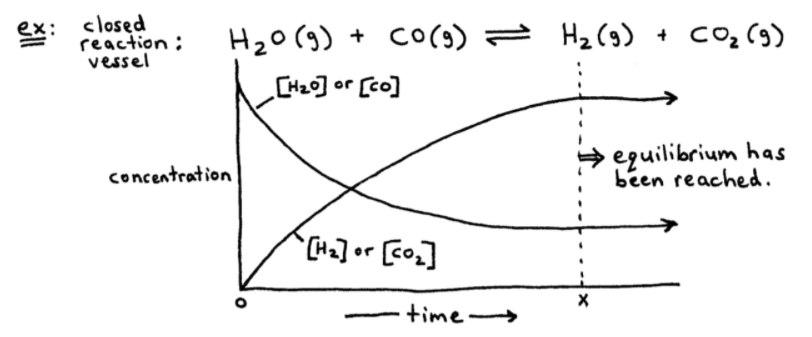# S13E1 - What is the Definition of 'Chemical Equilibrium' ?

Chemical Equilibrium
=  the state of a reaction in which the concentrations of reactants and products remain constant over time.

----------

## What is "Chemical Equilibrium"?

In this video we'll see how and why a chemical system comes to equilibrium.

We'll also discuss the characteristics of chemical equilibrium.

In later videos, we'll learn how to calculate the concentrations of reactants and products at equilibrium.

==========

## The Equilibrium Condition

While most reactions "go to completion" and proceed until the limiting reactant is consumed, some reactions stop far short of completion.

Chemical Equilibrium  =  a "dynamic" condition in which the forward and reverse reactions exactly offset each other, so no net reaction is occurs:➞  Right after time = 0 above, the concentrations of reactants decrease as the concentrations of products build up.

➞  After a certain period of time (x), equilibrium is reached.

➞  At time = x, or at equilibrium, enough [product] has been established.

➞  So now,

### Forward Reaction  =  Reverse Reaction

This is a dynamic equilibrium.

In the above example, equilibrium lies to the right because there are more products than reactants at time = x.

At equilibrium, [products] are greater in this example.

==========

## Dynamic Equilibrium Example

ex:  What happens if we inject some H2O(g) molecules into the system (the reaction) at equilibrium?
_________** If you suddently increase [H2O], more H2O is around, which means:

1.  more collisions between the H2O and CO reactant molecules,

2.  So... the forward reaction speeds up relative to the reverse reaction.

3.  Eventually however, the reverse reaction will "catch up" as more H2 and CO2 products are formed.

4.  Finally a new equilibrium is reached. The equilibrium has shifted to the right.

==========

This was just a short introduction to the beginning of SECTION 13 - Chemical Equilibrium.

In my next video post on this Section ("Chapter"),

We'll discuss the General Expressions for Chemical Equilibrium...